# Concentration function

(diff) ← Older revision | Latest revision (diff) | Newer revision → (diff)

of a random variableThe function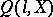defined for all non-negativeand random variablesby the formulaThe concentration functionis a non-negative, semi-additive, monotone decreasing function for, right continuous and such that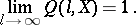Conversely, any function possessing these properties can be regarded as the concentration function of some random variable.

The concentration function is a convenient characterization of the scatter of the values of a random variable, especially for the quantitative expression of the increase of the scatter under summation of independent random variables. The first absolute (that is, containing only absolute constants) estimate for the concentration of the sum under given concentrations of the components was obtained by A.N. Kolmogorov  by developing a method due to P. Lévy . This result was subsequently strengthened (see ). The following statement was obtained, which includes all earlier results as special cases: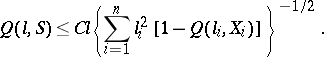Hereis a set of independent random variables,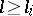,, andis an absolute constant. Two types of estimates are singled out: estimates ofof local type (see ), and estimates of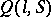of integral type (see ).

A dual characterization of the scatter, closely related to the concentration function, is the scattering function of the random variable: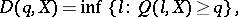where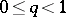. There is (see ) the following inequality relating the concentration function and the characteristic functionof a random variable: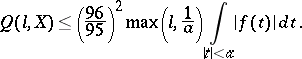The inequalitieswhere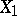andare independent random variables, also hold. There have been attempts to carry over certain results touching upon concentration functions to the case of summation of independent random vectors (see ).

How to Cite This Entry:
Concentration function. Encyclopedia of Mathematics. URL: http://encyclopediaofmath.org/index.php?title=Concentration_function&oldid=11867
This article was adapted from an original article by B.A. Rogozin (originator), which appeared in Encyclopedia of Mathematics - ISBN 1402006098. See original article